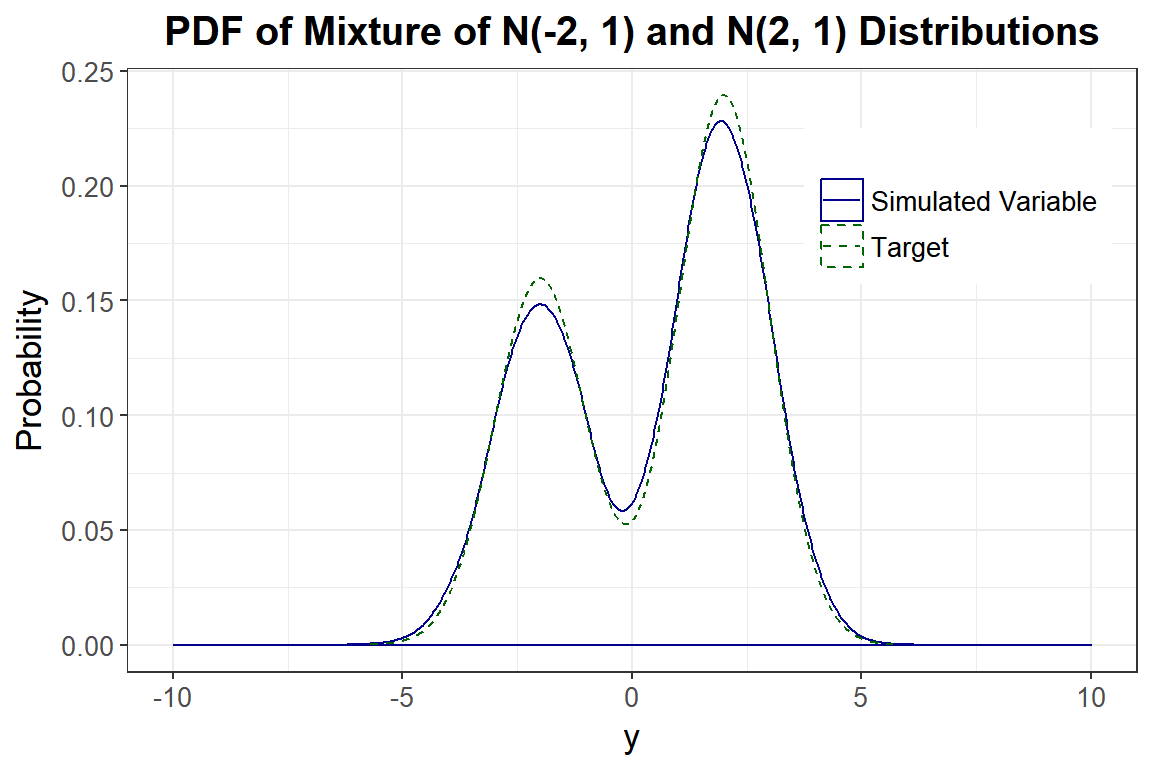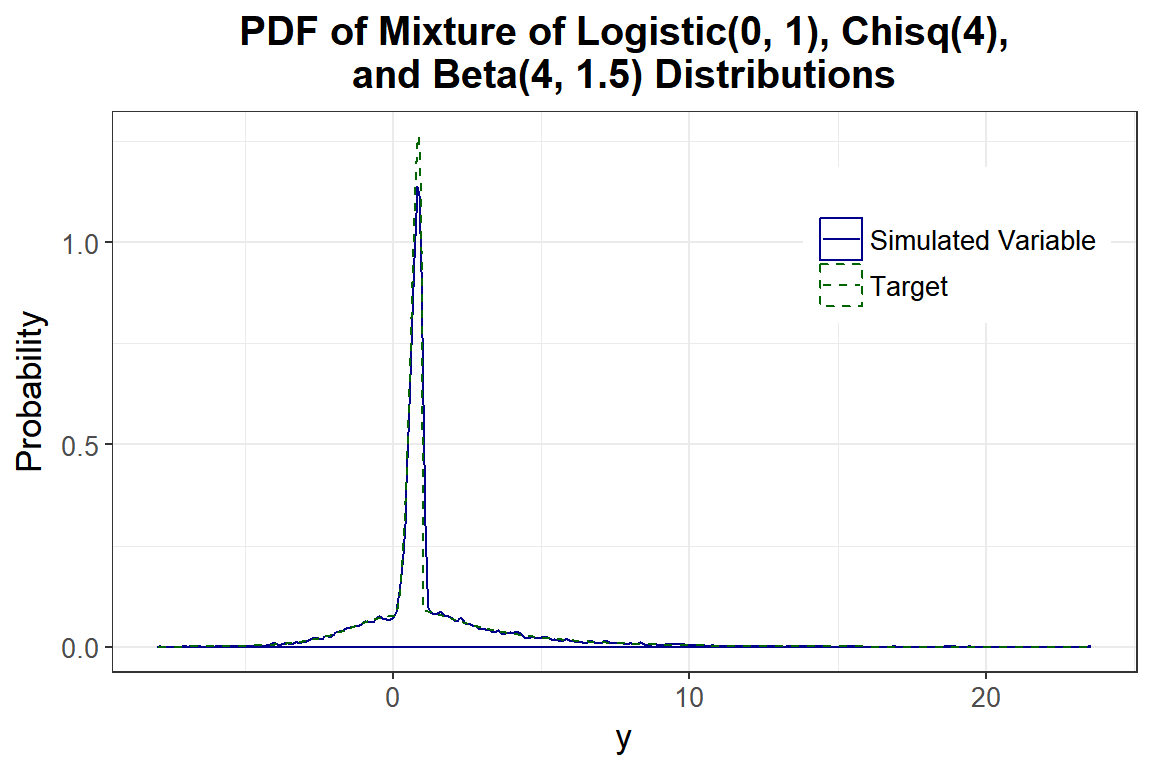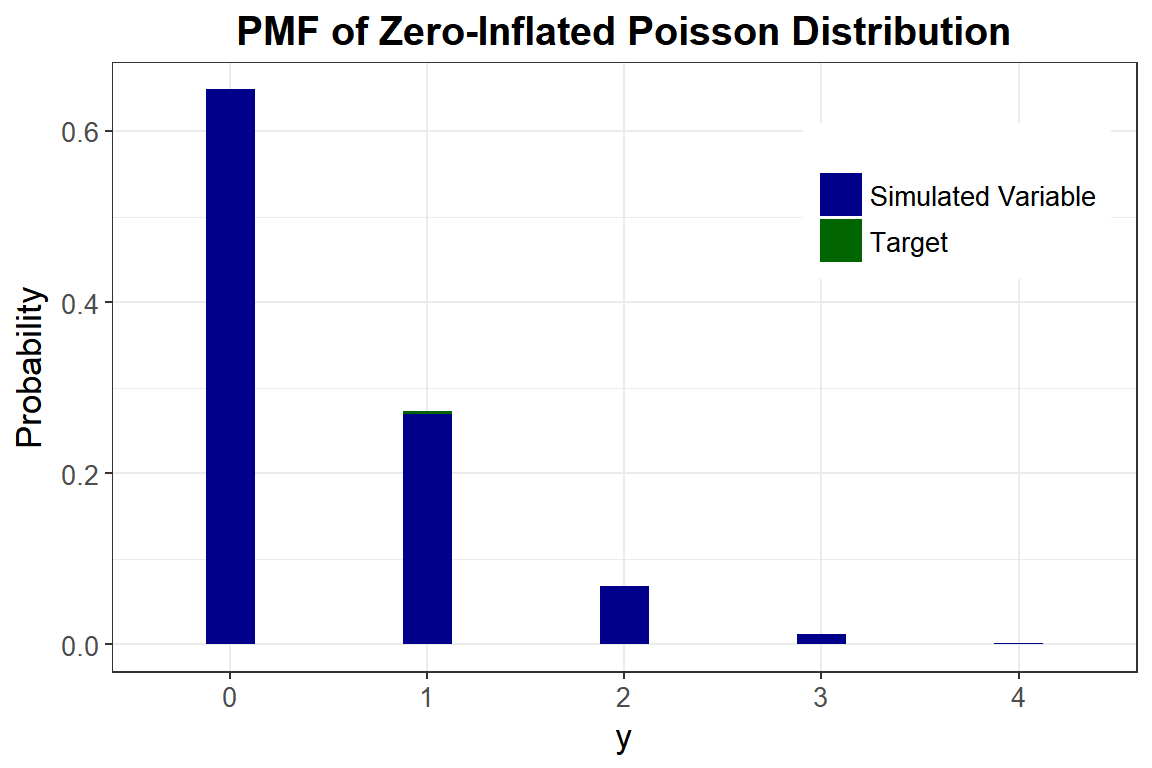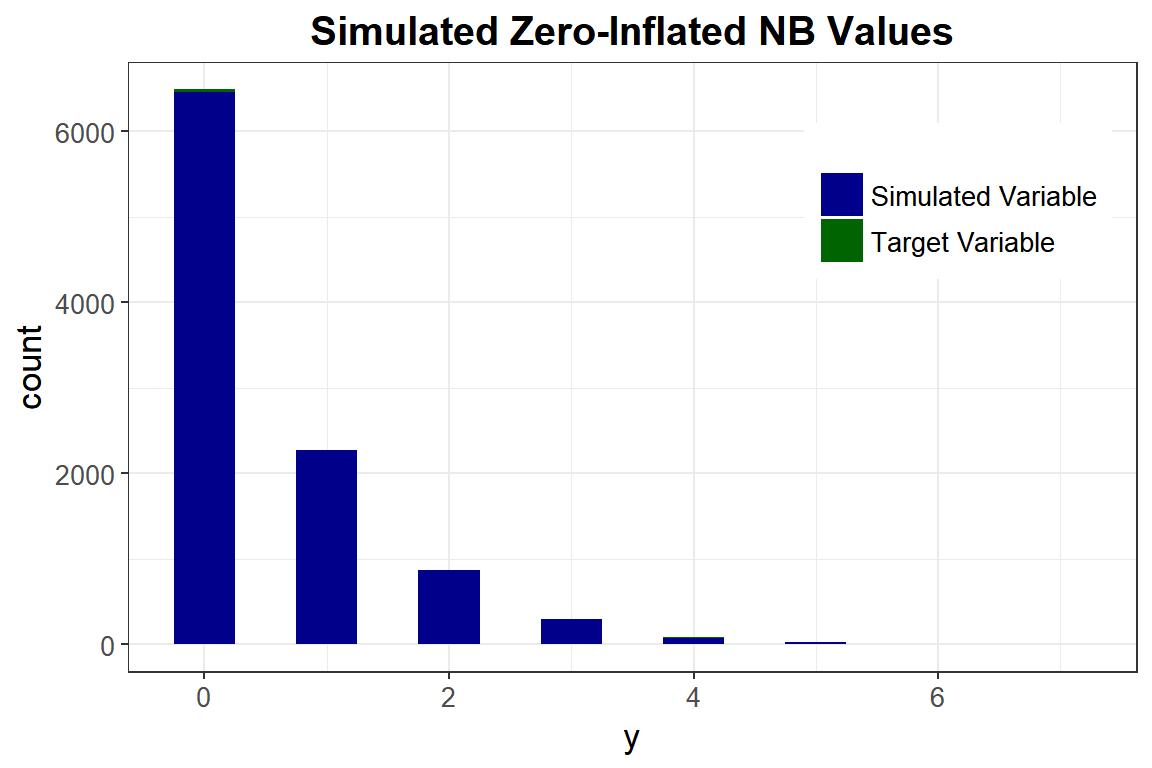This is a step-by-step guideline for correlated data simulation. More information about the different variable types can be found in the Variable Types vignette. More information about the differences between correlation methods 1 and 2 can be found in the Comparison of Correlation Methods 1 and 2 vignette. Some functions have been modified from the SimMultiCorrData package (Fialkowski 2018).

1. Obtain the distributional parameters for the desired variables.

1. Continuous variables: these are skew, standardized kurtosis (kurtosis - 3), and standardized fifth and sixth cumulants (for the fifth-order power method transformation, PMT). If the goal is to simulate a theoretical distribution (i.e. Gamma, Beta, Logistic, etc.), these values can be obtained using SimMultiCorrData::calc_theory. If the goal is to mimic an empirical data set, these values can be found using SimMultiCorrData::calc_moments (using the method of moments) or SimMultiCorrData::calc_fisherk (using Fisher’s k-statistics). If the standardized cumulants are obtained from calc_theory, the user may need to use rounded values as inputs (i.e. skews = round(skews, 8)). For example, in order to ensure that skew is exactly $$0$$ for symmetric distributions. Due to the nature of the integration involved in calc_theory, the results are approximations. Greater accuracy can be achieved by increasing the number of subdivisions (sub) used in the integration process.
1. For mixture variables, the parameters are specified at the component level by the inputs mix_skews, mix_skurts, mix_fifths, mix_sixths, and mix_Six. The mixing probabilities, means, and standard deviations of the component variables are given by mix_pis, mix_mus and mix_sigmas.

2. The means and variances of non-mixture and mixture variables are specified by means and vars. These are at the variable level, i.e., they refer to the continuous non-mixture and mixture variables themselves. The function calc_mixmoments calculates the expected mean, standard deviation, and standardized cumulants for mixture variables based on the component distributions.

3. For some sets of cumulants, it is either not possible to find power method constants or the calculated constants do not generate valid power method PDF’s. In these situations, adding a value to the sixth cumulant may provide solutions (see find_constants). If simulation results indicate that a continuous variable does not generate a valid PDF, the user can try find_constants with various sixth cumulant correction vectors to determine if a valid PDF can be found. These sixth cumulant corrections are specified in the simulation functions using Six or mix_Six.

4. Choice of Fleishman (1978)’s or Headrick (2002)’s Method: Using the fifth-order PMT (method = “Polynomial”) allows additional control over the fifth and sixth moments of the generated distribution, improving accuracy. In addition, the range of feasible standardized kurtosis ($$\gamma_{2}$$) values, given skew ($$\gamma_{1}$$) and standardized fifth ($$\gamma_{3}$$) and sixth ($$\gamma_{4}$$) cumulants, is larger than with the third-order method (method = “Fleishman”). For example, Fleishman’s method can not be used to generate a non-normal distribution with a ratio of $$\gamma_{1}^2/\gamma_{2} > 9/14$$ (Headrick and Kowalchuk 2007). This eliminates the family of distributions, which has a constant ratio of $$\gamma_{1}^2/\gamma_{2} = 2/3$$. The fifth-order method also generates more distributions with valid PDF’s, However, if the fifth and sixth cumulants do not exist, the third-order PMT should be used.

1. Ordinal variables ($$r \ge 2$$ categories): these are the cumulative marginal probabilities and support values (if desired). The probabilities should be combined into a list of length equal to the number of ordinal variables. The $$i^{th}$$ element is a vector of the cumulative probabilities defining the marginal distribution of the $$i^{th}$$ variable. If the variable can take $$r$$ values, the vector will contain $$r - 1$$ probabilities (the $$r^{th}$$ is assumed to be $$1$$). For binary variables, the user-supplied probability should be the probability of the $$1^{st}$$ (lower) support value. This would ordinarily be considered the probability of failure ($$q$$), while the probability of the $$2^{nd}$$ (upper) support value would be considered the probability of success ($$p = 1 - q$$). The support values should be combined into a separate list. The $$i^{th}$$ element is a vector containing the $$r$$ ordered support values. If not provided, the default is for the $$i^{th}$$ element to be the vector $$1, ..., r$$.

2. Poisson variables: the lambda (mean > 0) values should be given as a vector (see stats::dpois). For zero-inflated Poisson variables, the probability of a structural zero is specified in p_zip (see VGAM::dzipois). The default is p_zip = 0 for all variables. For correlation method 2, the total cumulative probability truncation values are specified in pois_eps, with the default of $$0.0001$$ for all variables. The order for parameters should be regular then zero-inflated for all inputs. The distribution functions are taken from the VGAM package (Yee 2018).

3. Negative Binomial variables: the sizes (target number of successes) and either the success probabilities or the means should be given as vectors (see stats::dnbinom). The variable represents the number of failures which occur in a sequence of Bernoulli trials before the target number of successes is achieved. For zero-inflated NB variables, the probability of a structural zero is specified in p_zinb (see VGAM::dzinegbin). The default is p_zinb = 0 for all variables. For correlation method 2, the total cumulative probability truncation values are specified in nb_eps, with the default of $$0.0001$$ for all variables. The order for parameters should be regular then zero-inflated for all inputs. The distribution functions are taken from the VGAM package (Yee 2018).

2. Check that all parameter inputs have the correct format using validpar. There are no checks within the correlation validation or simulation functions in order to decrease simulation time.

3. If continuous variables are desired, verify that the standardized kurtoses are greater than the lower skurtosis bounds. These bounds can be calculated using SimMultiCorrData::calc_lower_skurt, given the skewness (for method = “Fleishman”) and standardized fifth and sixth cumulants (for method = “Polynomial”) for each variable. Different seeds should be examined to see if a lower boundary can be found. If a lower bound produces power method constants that yield an invalid PDF, the user may wish to provide a Skurt vector of kurtosis corrections. In this case, calc_lower_skurt will attempt to find the smallest value that produces a kurtosis which yields a valid power method PDF. In addition, if method = “Polynomial”, a sixth cumulant correction vector (Six) may be used to facilitate convergence of the root-solving algorithm. Since this step can take considerable computation time, the user may instead wish to perform this check after simulation if any of the variables have invalid power method PDF’s.

4. Check if the target correlation matrix rho falls within the feasible correlation bounds, given the parameters for the desired distributions. The ordering of the variables in rho must be 1st ordinal, 2nd continuous non-mixture, 3rd components of continuous mixture variables, 4th regular Poisson, 5th zero-inflated Poisson, 6th regular NB, and 7th zero-inflated NB. These bounds can be calculated using either validcorr (correlation method 1) or validcorr2 (correlation method 2). Note that falling within these bounds does not guarantee that the target correlation can be achieved. However, the check can alert the user to pairwise correlations that obviously fall outside the bounds.

5. Generate the variables using either correlation method 1 and corrvar or correlation method 2 and corrvar2. The user may want to try both to see which gives a better approximation to the variables and correlation matrix. The accuracy and simulation time will vary by situation. In addition, the error loop can minimize the correlation errors in most situations. See the Error Loop Algorithm vignette for details about the error loop.

6. Summarize the results numerically. The functions corrvar and corrvar2 do not provide variable or correlation summaries in order to decrease simulation time. These can be obtained using summary_var, which gives summaries by variable type, the final correlation matrix, and the maximum error between the final and target correlation matrices. Additional summary functions include: SimMultiCorrData::sim_cdf_prob (to calculate a cumulative probability up to a given continuous y value), SimMultiCorrData::power_norm_corr (to calculate the correlation between a continuous variable and the generating standard normal variable), and SimMultiCorrData::stats_pdf (to calculate the $$100 \alpha \%$$ symmetric trimmed-mean, median, mode, and maximum height of a valid power method PDF).

7. Summarize the results graphically. Comparing the simulated data to the target distribution demonstrates simulation accuracy. The graphing functions provided in this package and the SimMultiCorrData package can be used to display simulated data values, PDF’s, or CDF’s. The target distributions (either by theoretical distribution name or given an empirical data set) can be added to the data value or PDF plots. Cumulative probabilities can be added to the CDF plots (for continuous variables).

Examples

The following examples demonstrate the use of the corrvar and corrvar2 functions to simulate the following correlated variables (n = 10,000):

1. Ordinal variable: $$O1$$ is binary with $$p = 0.3$$.

2. Continuous non-mixture variables: $$C1$$ and $$C2$$ have a Logistic(0, 1) distribution.

3. Continuous mixture variables:
1. $$M1$$ consists of $$M1_1$$ = Normal(-2, 1) and $$M1_2$$ = Normal(2, 1) with mixing probabilities $$0.4$$ and $$0.6$$.
2. $$M2$$ consists of $$M2_1$$ = Logistic(0,1), $$M2_2$$ = Chisq(4), and $$M2_3$$ = Beta(4, 1.5) with mixing probabilities $$0.3$$, $$0.2$$, and $$0.5$$.
1. Poisson variable: $$P1$$ with $$\lambda = 0.5$$ is a zero-inflated Poisson variable with the probability of a structural zero set at $$0.1$$.

2. Negative Binomial variable: $$NB1$$ with $$size = 2$$ and $$\mu = 2/3$$ is a zero-inflated NB variable with the probability of a structural zero set at $$0.2$$.

Headrick (2002)’s fifth-order transformation (method = “Polynomial”) is used for the continuous variables.

The target pairwise correlation is set at $$0.35$$ between $$O1$$, $$C1$$, $$C2$$, $$M1_1$$, $$M1_2$$, $$M2_1$$, $$M2_2$$, $$M2_3$$, $$P1$$, and $$NB1$$. The correlation between the components of the same mixture variable (i.e., $$M1_1$$ and $$M1_2$$) is set at $$0$$. Therefore, the correlation is controlled at the component level for the mixture variables. However, the expected correlations for the mixture variables can be approximated (see the Expected Cumulants and Correlations for Continuous Mixture Variables vignette).

Step 1: Obtain the distributional parameters

library("SimCorrMix")
library("printr")
options(scipen = 999)
seed <- 276
n <- 10000

# Continuous variables
L <- calc_theory("Logistic", c(0, 1))
C <- calc_theory("Chisq", 4)
B <- calc_theory("Beta", c(4, 1.5))

# Non-mixture variables
skews <- rep(L, 2)
skurts <- rep(L, 2)
fifths <- rep(L, 2)
sixths <- rep(L, 2)
Six <- list(1.75, 1.75)

# Mixture variables
mix_pis <- list(c(0.4, 0.6), c(0.3, 0.2, 0.5))
mix_mus <- list(c(-2, 2), c(L, C, B))
mix_sigmas <- list(c(1, 1), c(L, C, B))
mix_skews <- list(rep(0, 2), c(L, C, B))
mix_skurts <- list(rep(0, 2), c(L, C, B))
mix_fifths <- list(rep(0, 2), c(L, C, B))
mix_sixths <- list(rep(0, 2), c(L, C, B))
mix_Six <- list(list(NULL, NULL), list(1.75, NULL, 0.03))
Nstcum <- calc_mixmoments(mix_pis[], mix_mus[], mix_sigmas[],
mix_skews[], mix_skurts[], mix_fifths[], mix_sixths[])
Mstcum <- calc_mixmoments(mix_pis[], mix_mus[], mix_sigmas[],
mix_skews[], mix_skurts[], mix_fifths[], mix_sixths[])

means <- c(L, L, Nstcum, Mstcum)
vars <- c(L^2, L^2, Nstcum^2, Mstcum^2)

marginal <- list(0.3)
support <- list(c(0, 1))
lam <- 0.5
p_zip <- 0.1
size <- 2
prob <- 0.75
mu <- size * (1 - prob)/prob
p_zinb <- 0.2

k_cat <- length(marginal)
k_cont <- length(Six)
k_mix <- length(mix_pis)
k_comp <- sum(unlist(lapply(mix_pis, length)))
k_pois <- length(lam)
k_nb <- length(size)
k_total <- k_cat + k_cont + k_comp + k_pois + k_nb

Rey <- matrix(0.35, k_total, k_total)
diag(Rey) <- 1
rownames(Rey) <- colnames(Rey) <- c("O1", "C1", "C2", "M1_1", "M1_2", "M2_1",
"M2_2", "M2_3", "P1", "NB1")
Rey["M1_1", "M1_2"] <- Rey["M1_2", "M1_1"] <- 0
Rey["M2_1", "M2_2"] <- Rey["M2_2", "M2_1"] <- Rey["M2_1", "M2_3"] <-
Rey["M2_3", "M2_1"] <- Rey["M2_2", "M2_3"] <- Rey["M2_3", "M2_2"] <- 0

Step 2: Check the parameter inputs

validpar(k_cat, k_cont, k_mix, k_pois, k_nb, "Polynomial",
means, vars, skews, skurts, fifths, sixths, Six, mix_pis, mix_mus,
mix_sigmas, mix_skews, mix_skurts, mix_fifths, mix_sixths, mix_Six,
marginal, support, lam, p_zip, size, prob, mu = NULL, p_zinb, rho = Rey)
##  TRUE

Step 3: Calculate the lower skurtosis bounds for the continuous variables

Since this step takes considerable computation time, the user may wish to calculate these after simulation if any of the simulated continuous variables have invalid PDF’s. The calculation will be demonstrated for the Chisq(4) distribution using both the third and fifth-order PMT’s for comparison.

Using Fleishman (1978)’s third-order method:

Lower_third <- calc_lower_skurt(method = "Fleishman", skews = C,
Skurt = seq(1.161, 1.17, 0.001), seed = 104)
knitr::kable(Lower_third$Min[, c("skew", "valid.pdf", "skurtosis")], row.names = FALSE, caption = "Third-Order Lower Skurtosis Bound") Table 1: Third-Order Lower Skurtosis Bound skew valid.pdf skurtosis 1.414214 TRUE 3.141272 The original lower skurtosis boundary (see Lower_third$Invalid.C) of 1.979272 has been increased to 3.141272, so that the skurtosis correction is 1.162. The skurtosis for the distribution ($$3$$) is lower than this boundary and the third-order PMT should not be used to simulate this variable.

Using Headrick (2002)’s fifth-order method:

Lower_fifth <- calc_lower_skurt(method = "Polynomial", skews = C,
fifths = C, sixths = C, Skurt = seq(0.022, 0.03, 0.001), seed = 104)
knitr::kable(Lower_fifth$Min[, c("skew", "fifth", "sixth", "valid.pdf", "skurtosis")], row.names = FALSE, caption = "Fifth-Order Lower Skurtosis Bound") Table 2: Fifth-Order Lower Skurtosis Bound skew fifth sixth valid.pdf skurtosis 1.414214 8.485281 30 TRUE 2.998959 The original lower skurtosis boundary (see Lower_fifth$Invalid.C) of 2.975959 has been increased to 2.998959, so that the skurtosis correction is 0.023. The skurtosis for the distribution ($$3$$) is approximately equal to this boundary. This does not cause a problem since the simulated variable has a valid power method PDF.

The remaining steps vary by simulation method:

Simulation using Correlation Method 1:

Step 4: Verify the target correlation matrix falls within the feasible correlation bounds

valid1 <- validcorr(n, k_cat, k_cont, k_mix, k_pois, k_nb, "Polynomial",
means, vars, skews, skurts, fifths, sixths, Six, mix_pis, mix_mus,
mix_sigmas, mix_skews, mix_skurts, mix_fifths, mix_sixths, mix_Six,
marginal, lam, p_zip, size, prob, mu = NULL, p_zinb, Rey, seed)
## All correlations are in feasible range!

Step 5: Generate the variables

Sim1 <- corrvar(n, k_cat, k_cont, k_mix, k_pois, k_nb,
"Polynomial", means, vars, skews, skurts, fifths, sixths, Six,
mix_pis, mix_mus, mix_sigmas, mix_skews, mix_skurts, mix_fifths,
mix_sixths, mix_Six, marginal, support, lam, p_zip, size, prob,
mu = NULL, p_zinb, Rey, seed, epsilon = 0.01)
## Total Simulation time: 0.037 minutes

Step 6: Summarize the results numerically and Step 7: Summarize the results graphically

Sum1 <- summary_var(Sim1$Y_cat, Sim1$Y_cont, Sim1$Y_comp, Sim1$Y_mix,
Sim1$Y_pois, Sim1$Y_nb, means, vars, skews, skurts, fifths, sixths,
mix_pis, mix_mus, mix_sigmas, mix_skews, mix_skurts, mix_fifths,
mix_sixths, marginal, lam, p_zip, size, prob, mu = NULL, p_zinb, Rey)
Sim1_error <- abs(Rey - Sum1$rho_calc) Summary of correlation errors: summary(as.numeric(Sim1_error)) Min. 1st Qu. Median Mean 3rd Qu. Max. 0 0.0003912 0.0017661 0.0062285 0.0057543 0.0480139 Simulated correlation matrix for $$O1$$, $$C1$$, $$C2$$, $$M1$$, $$M2$$, $$P1$$, and $$NB1$$: rho_mix <- Sum1$rho_mix
rownames(rho_mix) <- c("01", "C1", "C2", "M1", "M2", "P1", "NB1")
colnames(rho_mix) <- rownames(rho_mix)
rho_mix
01 C1 C2 M1 M2 P1 NB1
01 1.0000000 0.3449509 0.3515655 0.1642942 0.1987437 0.3090497 0.3019861
C1 0.3449509 1.0000000 0.3499182 0.1566208 0.1926035 0.3532803 0.3521983
C2 0.3515655 0.3499182 1.0000000 0.1656771 0.2035505 0.3391623 0.3607957
M1 0.1642942 0.1566208 0.1656771 1.0000000 0.0859799 0.1717365 0.1569002
M2 0.1987437 0.1926035 0.2035505 0.0859799 1.0000000 0.2051287 0.2173914
P1 0.3090497 0.3532803 0.3391623 0.1717365 0.2051287 1.0000000 0.3626053
NB1 0.3019861 0.3521983 0.3607957 0.1569002 0.2173914 0.3626053 1.0000000

We can approximate the expected correlations using the formulas in the Expected Cumulants and Correlations for Continuous Mixture Variables vignette and the rho_M1M2 and rho_M1Y functions:

p_M11M21 <- p_M11M22 <- p_M11M23 <- 0.35
p_M12M21 <- p_M12M22 <- p_M12M23 <- 0.35
p_M1M2 <- matrix(c(p_M11M21, p_M11M22, p_M11M23, p_M12M21, p_M12M22, p_M12M23),
2, 3, byrow = TRUE)
rhoM1M2 <- rho_M1M2(mix_pis, mix_mus, mix_sigmas, p_M1M2)
1. The correlation between $$M1$$ and $$M2$$ is approximated as 0.0877342. The simulated correlation is 0.0859799.
p_M11C1 <- p_M12C1 <- 0.35
p_M1C1 <- c(p_M11C1, p_M12C1)
rho_M1C1 <- rho_M1Y(mix_pis[], mix_mus[], mix_sigmas[], p_M1C1)
1. The correlation between $$M1$$ and $$C1$$ is approximated as 0.1590909. The simulated correlation is 0.1566208.
p_M21C1 <- p_M22C1 <- p_M23C1 <- 0.35
p_M2C1 <- c(p_M21C1, p_M22C1, p_M23C1)
rho_M2C1 <- rho_M1Y(mix_pis[], mix_mus[], mix_sigmas[], p_M2C1)
1. The correlation between $$M2$$ and $$C1$$ is approximated as 0.1930151. The simulated correlation is 0.1926035.

Do all continuous variables have valid PDF’s?

Sim1$valid.pdf ##  "TRUE" "TRUE" "TRUE" "TRUE" "TRUE" "TRUE" "TRUE" Sim1$sixth_correction
##  1.75 1.75   NA   NA 1.75   NA 0.03

Non-mixture continuous variables and components of mixture variables:

target_sum <- Sum1$target_sum cont_sum <- Sum1$cont_sum
rownames(target_sum) <- rownames(cont_sum) <- c("C1", "C2", "M1_1", "M1_2",
"M2_1", "M2_2", "M2_3")
knitr::kable(target_sum, digits = 5, row.names = TRUE,
caption = "Summary of Target Distributions")
Table 3: Summary of Target Distributions
Distribution Mean SD Skew Skurtosis Fifth Sixth
C1 1 0.00000 3.28987 0.00000 1.2000 0.00000 6.85714
C2 2 0.00000 3.28987 0.00000 1.2000 0.00000 6.85714
M1_1 3 -2.00000 1.00000 0.00000 0.0000 0.00000 0.00000
M1_2 4 2.00000 1.00000 0.00000 0.0000 0.00000 0.00000
M2_1 5 0.00000 3.28987 0.00000 1.2000 0.00000 6.85714
M2_2 6 4.00000 8.00000 1.41421 3.0000 8.48528 30.00000
M2_3 7 0.72727 0.03051 -0.69388 -0.0686 1.82825 -3.37911
knitr::kable(cont_sum[, -c(2, 5:7)], digits = 5, row.names = TRUE,
caption = "Summary of Simulated Distributions")
Table 3: Summary of Simulated Distributions
Distribution Mean SD Skew Skurtosis Fifth Sixth
C1 1 -0.00287 1.81664 -0.12011 1.40786 -2.24058 11.41755
C2 2 -0.00235 1.81326 -0.07377 1.14818 -0.75267 5.28291
M1_1 3 -2.00000 0.99995 -0.00682 0.03108 -0.06622 0.06271
M1_2 4 2.00000 0.99995 -0.00946 0.00582 -0.08557 -0.05229
M2_1 5 0.00136 1.81194 0.08924 1.17706 1.85416 7.93594
M2_2 6 4.00055 2.84733 1.48311 3.42233 10.47408 36.91613
M2_3 7 0.72752 0.17529 -0.71763 -0.01946 1.81549 -3.72666

Mixture continuous variables:

target_mix <- Sum1$target_mix mix_sum <- Sum1$mix_sum
rownames(target_mix) <- rownames(mix_sum) <- c("M1", "M2")
knitr::kable(target_mix, digits = 5, row.names = TRUE,
caption = "Summary of Target Distributions")
Table 4: Summary of Target Distributions
Distribution Mean SD Skew Skurtosis Fifth Sixth
M1 1 0.40000 2.20000 -0.28850 -1.15402 1.79302 6.17327
M2 2 1.16364 2.17086 2.01328 8.78954 36.48103 192.72198
knitr::kable(mix_sum[, -c(2, 5:7)], digits = 5, row.names = TRUE,
caption = "Summary of Simulated Distributions")
Table 4: Summary of Simulated Distributions
Distribution Mean SD Skew Skurtosis Fifth Sixth
M1 1 0.40000 2.19989 -0.30573 -1.12584 1.86882 5.83223
M2 2 1.16364 2.17075 2.02355 9.04578 39.87345 223.50952
Nplot <- plot_simpdf_theory(sim_y = Sim1$Y_mix[, 1], ylower = -10, yupper = 10, title = "PDF of Mixture of N(-2, 1) and N(2, 1) Distributions", fx = function(x) mix_pis[] * dnorm(x, mix_mus[], mix_sigmas[]) + mix_pis[] * dnorm(x, mix_mus[], mix_sigmas[]), lower = -Inf, upper = Inf, sim_size = 0.5, target_size = 0.5) NplotMplot <- plot_simpdf_theory(sim_y = Sim1$Y_mix[, 2],
title = paste("PDF of Mixture of Logistic(0, 1), Chisq(4),",
"\nand Beta(4, 1.5) Distributions", sep = ""),
fx = function(x) mix_pis[] * dlogis(x, 0, 1) + mix_pis[] *
dchisq(x, 4) + mix_pis[] * dbeta(x, 4, 1.5),
lower = -Inf, upper = Inf, sim_size = 0.5, target_size = 0.5)
Mplotknitr::kable(Sum1$ord_sum, caption = "Summary of Ordinal Variables") Table 5: Summary of Ordinal Variables Target Simulated 0 0.3 0.298 1 1.0 1.000 knitr::kable(Sum1$pois_sum[, -c(2, 9:11)],
caption = "Summary of Poisson Variables")
Table 5: Summary of Poisson Variables
Distribution P0 Exp_P0 Mean Exp_Mean Var Exp_Var Skew Skurtosis
mean 1 0.6486 0.6458776 0.4498 0.45 0.48008 0.5277778 1.58424 2.460644
Pplot <- plot_simpdf_theory(sim_y = Sim1$Y_pois[, 1], title = "PMF of Zero-Inflated Poisson Distribution", Dist = "Poisson", params = c(lam, p_zip), cont_var = FALSE, col_width = 0.25) Pplotknitr::kable(Sum1$nb_sum[, -c(2, 10:12)],
caption = "Summary of Negative Binomial Variables")
Table 6: Summary of Negative Binomial Variables
Distribution P0 Exp_P0 Prob Mean Exp_Mean Var Exp_Var Skew Skurtosis
mean 1 0.6464 0.65 0.75 0.5353 0.5333333 0.7613539 0.7822222 1.954248 4.490933
NBplot <- plot_simtheory(sim_y = Sim1$Y_nb[, 1], title = "Simulated Zero-Inflated NB Values", binwidth = 0.5, Dist = "Negative_Binomial", params = c(size, mu, p_zinb), cont_var = FALSE) NBplotSimulation using Correlation Method 2: For this example, mu is used to describe $$NB1$$ instead of prob for demonstration purposes. Step 4: Verify the target correlation matrix falls within the feasible correlation bounds pois_eps <- 0.0001 nb_eps <- 0.0001 valid2 <- validcorr2(n, k_cat, k_cont, k_mix, k_pois, k_nb, "Polynomial", means, vars, skews, skurts, fifths, sixths, Six, mix_pis, mix_mus, mix_sigmas, mix_skews, mix_skurts, mix_fifths, mix_sixths, mix_Six, marginal, lam, p_zip, size, prob = NULL, mu, p_zinb, pois_eps, nb_eps, Rey, seed) ## All correlations are in feasible range! Step 5: Generate the variables Sim2 <- corrvar2(n, k_cat, k_cont, k_mix, k_pois, k_nb, "Polynomial", means, vars, skews, skurts, fifths, sixths, Six, mix_pis, mix_mus, mix_sigmas, mix_skews, mix_skurts, mix_fifths, mix_sixths, mix_Six, marginal, support, lam, p_zip, size, prob = NULL, mu, p_zinb, pois_eps, nb_eps, Rey, seed, epsilon = 0.01) ## Total Simulation time: 0.006 minutes Step 6: Summarize the results numerically and Step 7: Summarize the results graphically Sum2 <- summary_var(Sim2$Y_cat, Sim2$Y_cont, Sim2$Y_comp, Sim2$Y_mix, Sim2$Y_pois, Sim2$Y_nb, means, vars, skews, skurts, fifths, sixths, mix_pis, mix_mus, mix_sigmas, mix_skews, mix_skurts, mix_fifths, mix_sixths, marginal, lam, p_zip, size, prob = NULL, mu, p_zinb, Rey) Sim2_error <- abs(Rey - Sum2$rho_calc)

Summary of correlation errors:

summary(as.numeric(Sim2_error))
Min. 1st Qu. Median Mean 3rd Qu. Max.
0 0.0008558 0.0018499 0.0053624 0.0061373 0.0370054

Simulated correlation matrix for $$O1$$, $$C1$$, $$C2$$, $$M1$$, $$M2$$, $$P1$$, and $$NB1$$:

rho_mix <- Sum2$rho_mix rownames(rho_mix) <- c("01", "C1", "C2", "M1", "M2", "P1", "NB1") colnames(rho_mix) <- rownames(rho_mix) rho_mix 01 C1 C2 M1 M2 P1 NB1 01 1.0000000 0.3526467 0.3494859 0.1522525 0.1967726 0.3481883 0.3412737 C1 0.3526467 1.0000000 0.3477938 0.1564539 0.1878836 0.3481095 0.3561373 C2 0.3494859 0.3477938 1.0000000 0.1632542 0.1985422 0.3505951 0.3607467 M1 0.1522525 0.1564539 0.1632542 1.0000000 0.0907563 0.1539732 0.1692886 M2 0.1967726 0.1878836 0.1985422 0.0907563 1.0000000 0.2018016 0.1964330 P1 0.3481883 0.3481095 0.3505951 0.1539732 0.2018016 1.0000000 0.3495915 NB1 0.3412737 0.3561373 0.3607467 0.1692886 0.1964330 0.3495915 1.0000000 Do all continuous variables have valid PDF’s? Sim2$valid.pdf
##  "TRUE" "TRUE" "TRUE" "TRUE" "TRUE" "TRUE" "TRUE"
Sim2$sixth_correction ##  1.75 1.75 NA NA 1.75 NA 0.03 Non-mixture continuous variables and components of mixture variables: target_sum <- Sum2$target_sum
cont_sum <- Sum2$cont_sum rownames(target_sum) <- rownames(cont_sum) <- c("C1", "C2", "M1_1", "M1_2", "M2_1", "M2_2", "M2_3") knitr::kable(target_sum, digits = 5, row.names = TRUE, caption = "Summary of Target Distributions") Table 7: Summary of Target Distributions Distribution Mean SD Skew Skurtosis Fifth Sixth C1 1 0.00000 3.28987 0.00000 1.2000 0.00000 6.85714 C2 2 0.00000 3.28987 0.00000 1.2000 0.00000 6.85714 M1_1 3 -2.00000 1.00000 0.00000 0.0000 0.00000 0.00000 M1_2 4 2.00000 1.00000 0.00000 0.0000 0.00000 0.00000 M2_1 5 0.00000 3.28987 0.00000 1.2000 0.00000 6.85714 M2_2 6 4.00000 8.00000 1.41421 3.0000 8.48528 30.00000 M2_3 7 0.72727 0.03051 -0.69388 -0.0686 1.82825 -3.37911 knitr::kable(cont_sum[, -c(2, 5:7)], digits = 5, row.names = TRUE, caption = "Summary of Simulated Distributions") Table 7: Summary of Simulated Distributions Distribution Mean SD Skew Skurtosis Fifth Sixth C1 1 0.00137 1.81558 0.06184 1.32049 1.15791 11.32372 C2 2 -0.00316 1.81408 -0.05852 1.20886 0.33438 6.66564 M1_1 3 -2.00000 0.99995 0.01645 0.03766 -0.14312 0.11787 M1_2 4 2.00000 0.99995 -0.00513 -0.00950 0.04604 0.03334 M2_1 5 0.00114 1.81206 0.08295 1.16386 1.84537 7.37171 M2_2 6 4.00044 2.84502 1.46747 3.27444 9.44722 30.91024 M2_3 7 0.72749 0.17520 -0.71409 -0.02244 1.79531 -3.66029 Mixture continuous variables: target_mix <- Sum2$target_mix
mix_sum <- Sum2$mix_sum rownames(target_mix) <- rownames(mix_sum) <- c("M1", "M2") knitr::kable(target_mix, digits = 5, row.names = TRUE, caption = "Summary of Target Distributions") Table 8: Summary of Target Distributions Distribution Mean SD Skew Skurtosis Fifth Sixth M1 1 0.40000 2.20000 -0.28850 -1.15402 1.79302 6.17327 M2 2 1.16364 2.17086 2.01328 8.78954 36.48103 192.72198 knitr::kable(mix_sum[, -c(2, 5:7)], digits = 5, row.names = TRUE, caption = "Summary of Simulated Distributions") Table 8: Summary of Simulated Distributions Distribution Mean SD Skew Skurtosis Fifth Sixth M1 1 0.40000 2.19989 -0.30025 -1.13041 1.85623 5.88668 M2 2 1.16364 2.17075 2.00831 8.85771 37.06375 191.28657 knitr::kable(Sum2$ord_sum, caption = "Summary of Ordinal Variables")
Table 9: Summary of Ordinal Variables
Target Simulated
0 0.3 0.3007
1 1.0 1.0000
knitr::kable(Sum2$pois_sum[, -c(2, 9:11)], caption = "Summary of Poisson Variables") Table 9: Summary of Poisson Variables Distribution P0 Exp_P0 Mean Exp_Mean Var Exp_Var Skew Skurtosis mean 1 0.6468 0.6458776 0.4504 0.45 0.4749398 0.5277778 1.554168 2.331026 knitr::kable(Sum2$nb_sum[, -c(2, 10:12)],
caption = "Summary of Negative Binomial Variables")
Table 10: Summary of Negative Binomial Variables
Distribution P0 Exp_P0 Prob Mean Exp_Mean Var Exp_Var Skew Skurtosis
mean 1 0.6509 0.65 0.75 0.5342 0.5333333 0.7904304 0.7822222 2.084995 5.33719